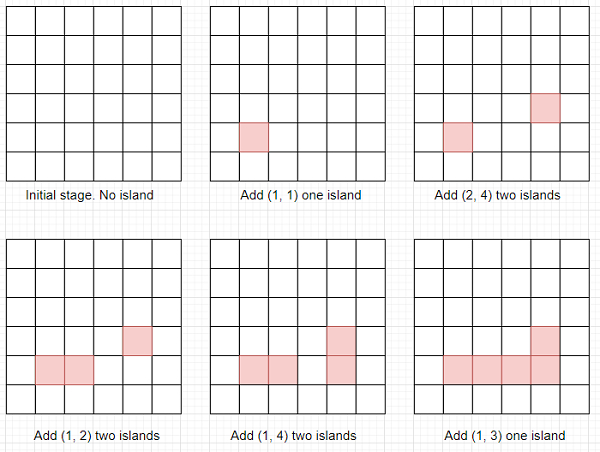# Program to find island count by adding blocks into grid one by one in Python

Suppose we have one infinite grid of water. We can add blocks of land to that grid one by one. We have a list of coordinates called land_requests where each coordinate is in the form [r, c] where r is for row and c is for column. We have to find a list where each element represents the number of islands that exist after adding each block of land from land_requests.

So, if the input is like land_requests = [[1, 1],[2, 4],[1, 2],[1, 4],[1, 3]], then the output will be [1, 2, 2, 2, 1] asTo solve this, we will follow these steps −

• d := a list of directions like [(−1, 0) ,(0, 1) ,(1, 0) ,(0, −1) ]

• idx := 0

• mp := a new map

• p := a new list

• size := a new list

• comp := 0

• ans := a new list

• Define a function search() . This will take u

• if u is same as p[u], then

• return u

• p[u] := search(p[u])

• return p[u]

• Define a function connect() . This will take u, v

• pu := search(u), pv := search(v)

• if pu is same as pv, then

• return

• comp := comp − 1

• if size[pu] >= size[pv], then

• p[pv] := pu

• size[pu] := size[pu] + size[pv]

• otherwise,

• p[pu] := pv

• size[pv] := size[pv] + size[pu]

• From the main method do the following −

• for each request in land_requests, do

• (i, j) := request

• mp[i, j] := idx

• insert idx at the end of p

• insert 1 at the end of size

• idx := idx + 1

• comp := comp + 1

• for each k in d, do

• ni := i + k

• nj := j + k

• if (ni, nj) is in mp, then

• connect(mp[(i, j)], mp[(ni, nj)])

• insert comp at the end of ans

• return ans

Let us see the following implementation to get better understanding −

## Example

Live Demo

d = [(−1, 0), (0, 1), (1, 0), (0, −1)]
class Solution:
def search(self, u):
if u == self.p[u]:
return u
self.p[u] = self.search(self.p[u])
return self.p[u]
def connect(self, u, v):
pu = self.search(u)
pv = self.search(v)
if pu == pv:
return
self.comp −= 1
if self.size[pu] >= self.size[pv]:
self.p[pv] = pu
self.size[pu] += self.size[pv]
else:
self.p[pu] = pv
self.size[pv] += self.size[pu]
def solve(self, land_requests):
self.idx = 0
self.mp = dict()
self.p = []
self.size = []
self.comp = 0
ans = []
for request in land_requests:
i, j = request
self.mp[(i, j)] = self.idx
self.p.append(self.idx)
self.size.append(1)
self.idx += 1
self.comp += 1
for k in d:
ni = i + k
nj = j + k
if (ni, nj) in self.mp:
self.connect(self.mp[(i, j)], self.mp[(ni, nj)])
ans.append(self.comp)
return ans
ob = Solution()
land_requests = [[1, 1],[2, 4],[1, 2],[1, 4],[1, 3]]
print(ob.solve(land_requests))

## Input

[[1, 1],[2, 4],[1, 2],[1, 4],[1, 3]]

## Output

[1, 2, 2, 2, 1]